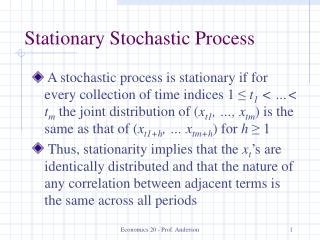DownloadDownload PresentationStationary Stochastic Process

# Stationary Stochastic Process

Télécharger la présentation## Stationary Stochastic Process

- - - - - - - - - - - - - - - - - - - - - - - - - - - E N D - - - - - - - - - - - - - - - - - - - - - - - - - - -
##### Presentation Transcript

1. Stationary Stochastic Process • A stochastic process is stationary if for every collection of time indices 1 ≤ t1 < …< tm the joint distribution of (xt1, …, xtm) is the same as that of (xt1+h, … xtm+h) for h≥ 1 • Thus, stationarity implies that the xt’s are identically distributed and that the nature of any correlation between adjacent terms is the same across all periods Economics 20 - Prof. Anderson

2. Covariance Stationary Process • A stochastic process is covariance stationary if E(xt) is constant, Var(xt) is constant and for any t, h≥ 1, Cov(xt, xt+h) depends only on h and not on t • Thus, this weaker form of stationarity requires only that the mean and variance are constant across time, and the covariance just depends on the distance across time Economics 20 - Prof. Anderson

3. Weakly Dependent Time Series • A stationary time series is weakly dependent if xtand xt+h are “almost independent” as h increases • If for a covariance stationary process Corr(xt, xt+h) → 0 as h → ∞, we’ll say this covariance stationary process is weakly dependent • Want to still use law of large numbers Economics 20 - Prof. Anderson

4. An MA(1) Process • A moving average process of order one [MA(1)] can be characterized as one where xt = et + a1et-1, t = 1, 2, … with et being an iid sequence with mean 0 and variance s2e • This is a stationary, weakly dependent sequence as variables 1 period apart are correlated, but 2 periods apart they are not Economics 20 - Prof. Anderson

5. An AR(1) Process • An autoregressive process of order one [AR(1)] can be characterized as one where yt = ryt-1 + et , t = 1, 2,… with et being an iid sequence with mean 0 and variance se2 • For this process to be weakly dependent, it must be the case that |r| < 1 • Corr(yt ,yt+h) = Cov(yt ,yt+h)/(sysy) = r1h which becomes small as h increases Economics 20 - Prof. Anderson

6. Trends Revisited • A trending series cannot be stationary, since the mean is changing over time • A trending series can be weakly dependent • If a series is weakly dependent and is stationary about its trend, we will call it a trend-stationary process • As long as a trend is included, all is well Economics 20 - Prof. Anderson

7. Assumptions for Consistency • Linearity and Weak Dependence • A weaker zero conditional mean assumption: E(ut|xt) = 0, for each t • No Perfect Collinearity • Thus, for asymptotic unbiasedness (consistency), we can weaken the exogeneity assumptions somewhat relative to those for unbiasedness Economics 20 - Prof. Anderson

8. Large-Sample Inference • Weaker assumption of homoskedasticity: Var (ut|xt) = s2, for each t • Weaker assumption of no serial correlation: E(utus| xt, xs) = 0 for t  s • With these assumptions, we have asymptotic normality and the usual standard errors, t statistics, F statistics and LM statistics are valid Economics 20 - Prof. Anderson

9. Random Walks • A random walk is an AR(1) model where r1 = 1, meaning the series is not weakly dependent • With a random walk, the expected value of yt is always y0 – it doesn’t depend on t • Var(yt) = se2t, so it increases with t • We say a random walk is highly persistent since E(yt+h|yt) = yt for all h≥ 1 Economics 20 - Prof. Anderson

10. Random Walks (continued) • A random walk is a special case of what’s known as a unit root process • Note that trending and persistence are different things – a series can be trending but weakly dependent, or a series can be highly persistent without any trend • A random walk with drift is an example of a highly persistent series that is trending Economics 20 - Prof. Anderson

11. Transforming Persistent Series • In order to use a highly persistent series and get meaningful estimates and make correct inferences, we want to transform it into a weakly dependent process • We refer to a weakly dependent process as being integrated of order zero, [I(0)] • A random walk is integrated of order one, [I(1)], meaning a first difference will be I(0) Economics 20 - Prof. Anderson